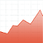V-Lab

## Motivation

Prior to the financial crisis of 2007 and 2008, standard risk management focused primarily on short-run risks such as 1-day value at risk ($\mathrm{VaR}$) or 10-day $\mathrm{VaR}$. Engle (2009) points out that most assets in portfolios are held longer than these horizons, and as such longer term risk measures are important from a risk management perspective. For example, in periods of low volatility excess leverage likely carries little short-run risk. However, volatility is mean reverting and will rise in the long run; hence, any short-term risk measure will understate the risk of a leveraged portfolio. The Long Run $\mathrm{VaR}$ section of V-Lab is designed to account for mean-reversion of volatility and build $\mathrm{VaR}$ models that account for “the risk that risk will change”.

## Definition

Consider an asset’s log-return series ${r}_{t}=\mu +{\epsilon }_{t}$, where $\mu$ is the expected return and ${\epsilon }_{t}$ is a zero-mean white noise. The total log-return between date $t$ and date $t+k$ is then naturally defined as:$rt,t+k=∑i=1krt+k$The standard definition of the $k$-day ahead $\mathrm{VaR}$ of a position in this asset is the 1% or 5% quantile of the return distribution for ${r}_{t,t+k}$. V-Lab’s long run risk measures use simulation based methods to calculate the $\mathrm{VaR}$ at horizons of $k=30$ and $k=365$.

## Esimation

One way to calculate $\mathrm{VaR}$ is to simulate future realizations of the return process and use the resulting simulations to calculate $\mathrm{VaR}$. For both of the models prescribed in the models section of the documentation, a volatility model is fit to historical data on each day. The resulting model is then simulated ahead 10,000 times, for a horizon of 1-year in advance. All simulations are bootstrapped - that is historical shocks to the return process are drawn at random to simulate each path. $\mathrm{VaR}$ is then calculated using both the 1% and 5% quantile of the 10,000 simulated return paths. Finally, logarithmic returns are converted back to arithmetic returns.

## References

Engle, Robert F., The Risk that Risk Will Change. Journal Of Investment Management (JOIM), Fourth Quarter 2009. https://www.joim.com/article/the-risk-that-risk-will-change/

Engle, R. F. and J. G. Rangel, 2008. The Spline-GARCH Model for Low-Frequency Volatility and Its Global Macroeconomic Causes. Review of Financial Studies 21(3): 1187-1222. https://www.jstor.org/stable/40056848

Glosten, L. R., R. Jagannathan, and D. E. Runkle, 1993. On The Relation between The Expected Value and The Volatility of Nominal Excess Return on stocks. Journal of Finance 48: 1779-1801. https://doi.org/10.1111/j.1540-6261.1993.tb05128.x

Zakoian, J. M., 1994. Threshold Heteroscedastic Models. Journal of Economic Dynamics and Control 18: 931-955. https://doi.org/10.1016/0165-1889(94)90039-6